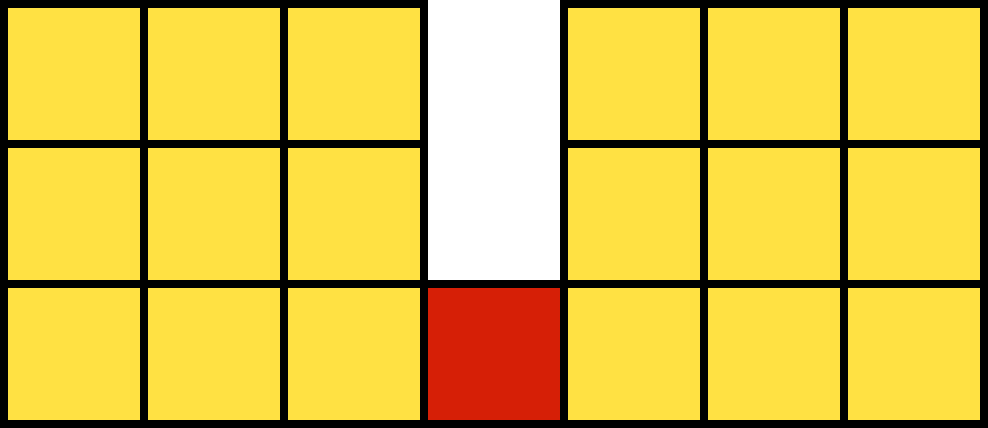# Rectangles

Probability Level 2The figure above consists of 19 unit squares. How many rectangles are there in the figure?


Note: Squares are also considered as rectangles.

×